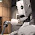## Wednesday, February 22, 2012

### A Python Implementation of The Log-Polar Transform, ReduxOne of my first posts to this blog presented a Python implementation of the log-polar transform. Back then I was just beginning to learn NumPy, and didn't know many of its more useful tricks; in particular, I had no domain of fancy indexing, and did not know that it could be used to avoid the need for time-consuming Python loops. Besides that, I later realized that the algorithm I chose to implement, taken from an article I had read at the time, was unnecessarily complicated.

A couple of months ago I was considering the use of the log-polar transform for another project, and thought I should go back and rewrite my early implementation. I wanted to make use of the NumPy expertise I've earned since then, but also clean up my early blunder and present a simpler algorithm, that could be easily ported to other languages. The results are available below, and also for download in a nice package, with test code and thoroughly commented sources.

The first listing presents a "naive" implementation, which relies on Python loops:
def logpolar_naive(image, i_0, j_0, p_n=None, t_n=None):
(i_n, j_n) = image.shape[:2]

i_c = max(i_0, i_n - i_0)
j_c = max(j_0, j_n - j_0)
d_c = (i_c ** 2 + j_c ** 2) ** 0.5

if p_n == None:
p_n = int(ceil(d_c))

if t_n == None:
t_n = j_n

p_s = log(d_c) / p_n
t_s = 2.0 * pi / t_n

transformed = zeros((p_n, t_n) + image.shape[2:], dtype=image.dtype)

for p in range(0, p_n):
p_exp = exp(p * p_s)
for t in range(0, t_n):
t_rad = t * t_s

i = int(i_0 + p_exp * sin(t_rad))
j = int(j_0 + p_exp * cos(t_rad))

if 0 <= i < i_n and 0 <= j < j_n:
transformed[p, t] = image[i, j]

return transformed

The second version constructs a pair of NumPy fancy indices first – cached for later reference – and then uses them to perform the transform:
_transforms = {}

def _get_transform(i_0, j_0, i_n, j_n, p_n, t_n, p_s, t_s):
transform = _transforms.get((i_0, j_0, i_n, j_n, p_n, t_n))

if transform == None:
i_k = []
j_k = []
p_k = []
t_k = []

for p in range(0, p_n):
p_exp = exp(p * p_s)
for t in range(0, t_n):
t_rad = t * t_s

i = int(i_0 + p_exp * sin(t_rad))
j = int(j_0 + p_exp * cos(t_rad))

if 0 <= i < i_n and 0 <= j < j_n:
i_k.append(i)
j_k.append(j)
p_k.append(p)
t_k.append(t)

transform = ((array(p_k), array(t_k)), (array(i_k), array(j_k)))
_transforms[i_0, j_0, i_n, j_n, p_n, t_n] = transform

return transform

def logpolar_fancy(image, i_0, j_0, p_n=None, t_n=None):
(i_n, j_n) = image.shape[:2]

i_c = max(i_0, i_n - i_0)
j_c = max(j_0, j_n - j_0)
d_c = (i_c ** 2 + j_c ** 2) ** 0.5

if p_n == None:
p_n = int(ceil(d_c))

if t_n == None:
t_n = j_n

p_s = log(d_c) / p_n
t_s = 2.0 * pi / t_n

(pt, ij) = _get_transform(i_0, j_0, i_n, j_n, p_n, t_n, p_s, t_s)

transformed = zeros((p_n, t_n) + image.shape[2:], dtype=image.dtype)

transformed[pt] = image[ij]
return transformed

Both implementations are based on a "reverse transform" algorithm: rather than iterate over the Cartesian image calculating where each pixel fits in the transform (which resulted in all sorts of complications), iterate over the transform's cells and calculate which, if any, pixel from the Cartesian image should be put there.

According to my tests, the "fancy" version using a cached pair of indices runs about 10 times faster than the "naive" version – but even when it has to construct the indices before using them, it's still slightly faster.

#### 3 comments:

1.Thank you for this code, this is very useful and clear.
Would you happen to already have coded the inverse function? I mean from logpolar to cartesian coordinates. Thanks anyway, and well done.

2.Not really, no. But it shouldn't be difficult to do it using the same solution as the forward transform – only now you'd iterate over cells on the Cartesian transform, calculating the indices of the corresponding cells in the Log-polar image and taking its values (or leaving the destination cells alone, wherever corresponding log-polar coordinates fell off the bounds of the source image).

3.I see, hurray for conformal maps. Thanks for a quick reply.